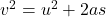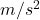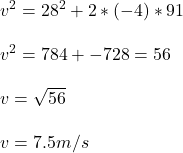## A speedboat moving at 28 m/s approaches a no-wake buoy marker 91 m ahead. The pilot slows the boat with a constant acceleration of 4 m/s2 by

Question

A speedboat moving at 28 m/s approaches a no-wake buoy marker 91 m ahead. The pilot slows the boat with a constant acceleration of 4 m/s2 by reducing the throttle. What is the velocity (in m/s) of the boat when it reaches the buoy

in progress 0
3 weeks 2021-08-26T04:57:32+00:00 1 Answers 0 views 0

7.5 m/s

Explanation:

We can find its velocity when it reaches the buoy by applying one of Newton’s equations of motion:where v = final velocity

u = initial velocity

a = acceleration

s = distance traveled

From the question:

u = 28 m/s

a = -4s = 91 m

Therefore:The velocity of the boat when it reaches the buoy is 7.5 m/s.